# Class 10 RD Sharma Solutions – Chapter 4 Triangles – Exercise 4.7 | Set 1

### Question 1. If the sides of a triangle are 3 cm, 4 cm, and 6 cm long, determine whether the triangle is a right-angled triangle.

Solution:

According to the question

The sides of triangle are:

AB = 3 cm

BC = 4 cm

AC = 6 cm

According to Pythagoras Theorem:

AB2 = 32 = 9

BC2 = 42 = 16

AC2 = 62 = 36

Since, AB2 + BC2 ≠ AC2

Hence, by converse of Pythagoras theorem, triangle is not a right triangle

### Question 2. The sides of certain triangles are given below. Determine which of them right triangles are.

(i) a = 7 cm, b = 24 cm and c = 25 cm

(ii) a = 9 cm, b = 16 cm and c = 18 cm

(iii) a = 1.6 cm, b = 3.8 cm and c = 4 cm

(iv) a = 8 cm, b = 10 cm and c = 6 cm

Solution:

(i) According to the question

The sides of triangle are:

a = 7 cm, b = 24 cm and c = 25 cm

According to Pythagoras Theorem:

The hypotenuse or longest side of a right angle triangle is equal to the sum of

squares of other two sides of the triangle

∴ a2 = 49, b2 = 576 and c2 = 625

(Longest side)2 = c2 = 625

Sum of squares of shorter sides = (a)2 + (b)2= 49 + 576 = 625

∴ 625 = 625

Hence, by converse of Pythagoras theorem, given triangle is a right triangle.

(ii) According to the question

The sides of triangle are: a = 9 cm, b = 16 cm and c = 18 cm

According to Pythagoras Theorem:

The hypotenuse or longest side of a right angle triangle is equal to the sum of

squares of other two sides of the triangle

∴ a2 = 81, b2 = 256 and c2 = 324

(Longest side)2 = c2 = 324

Sum of squares of shorter sides = (a)2 + (b)2 = 81 + 256 = 337

∴324 ≠ 337

Hence, by converse of Pythagoras theorem, given triangle is not a right triangle.

(iii) According to the question

The sides of triangle are: a = 1.6 cm, b = 3.8 cm and C = 4 cm

According to Pythagoras Theorem:

The hypotenuse or longest side of a right angle triangle is equal to the sum of

squares of other two sides of the triangle

(Longest side)2 = 16

Sum of squares of shorter sides = (1.6)2 + (3.8)2 = 2.56 + 14.44 = 17

∴ 16 ≠ 17

Hence, by converse of Pythagoras theorem, given triangle is not a light triangle.

(iv) According to the question

The sides of triangle are: a = 8 cm, b = 10 cm, c = 6 cm

According to Pythagoras Theorem:

The hypotenuse or longest side of a right angle triangle is equal to the sum of

squares of other two sides of the triangle

(Longest side)2 = (10)2 = 100

Sum of squares of shorter sides = (8)2 + (6)2 = 64 + 36 = 100

∴ 100 = 100

Hence, by converse of Pythagoras theorem, given triangle is a light triangle.

### Question 3. A man goes 15m due west and then 8m due north. How far is lie from the starting point?

Solution: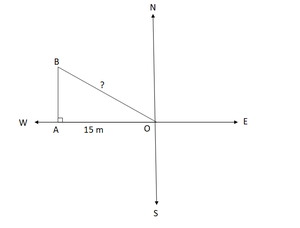Let the starting point of the man be O and final point be A.

So, In ∆ABO,

By using Pythagoras theorem

AO2 = AB2 + BO2

⟹ AO2 = 82 + 152

⟹ AO2 = 64 + 225 = 289

⟹ AO = √289 = 17m

Hence, the man is 17m far from the starting point.

### Question 4. A ladder 17 m long reaches a window of a building 15 m above the ground. Find the distance of the foot of the ladder from the building.

Solution: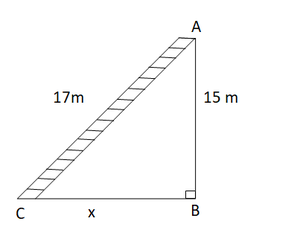In ∆ABC,

By using Pythagoras theorem

AB2 + BC2 = AC2

⟹ 152 + BC2 = 172

⟹ 225 + BC2 = 172

⟹ BC2 = 289 – 225

⟹ BC2 = 64

⟹ BC = 8 m

Hence, the distance of the foot of the ladder from building = 8 m

### Question 5. Two poles of heights 6 m and 11 m stand on a plane ground. If the distance between their feet is 12 m, find the distance between their tops.

Solution: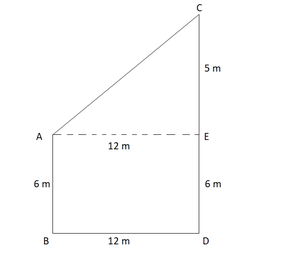Let us considered CD and AB be the poles of height 11 and 6 in.

Therefore, CP = 11 – 6 = 5 m

From the figure we may observe that AP = 12m

In ∆APC,

By using Pythagoras theorem

AP2 + PC2 = AC2

122 + 52 = AC2

AC2 = 144 + 25 = 169 m

AC = 13 m

Hence, the distance between their tops = 13 m.

### Question 6. In an isosceles triangle ABC. AB = AC = 25 cm, BC = 14 cm. Calculate the altitude from A on BC.

Solution: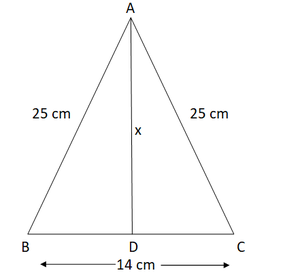According to the question

AB = AC = 25 cm and BC = 14

In ∆ABD and ∆ACD

AB = AC                              [Each = 25 cm]

So, by RHS condition

∆ABD ≅ ∆ACD

Hence, by corresponding parts of congruent triangles

∴ BD = CD = 7 cm

By using Pythagoras theorem

⟹ AD2 + 72 = 252

⟹ AD2 = 625 – 49 = 576

⟹ AD = √576 = 24 cm

### Question 7. The foot of a ladder is 6 m away from a wall and its top reaches a window 8 m above the ground. If the ladder is shifted in such a way that its foot is 8 m away from the wall, to what height does its tip reach?

Solution: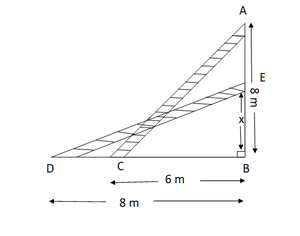In ∆ACD,

By using Pythagoras theorem

⟹ l2 = 82 + 62 … (i)

In ∆BCE,

By using Pythagoras theorem

BE2 = BC2 + CE

⟹ l2 = BC2 + 82 … (ii)

From eq(i) and (ii), we get

BC2 + 82 = 82 + 62

⟹ BC2 + 62

⟹ BC = 6 m

### Question 8. Two poles of height 9 m in and 14 m stand on a plane ground. If the distance between their feet is 12 m in, find the distance between their tops.

Solution: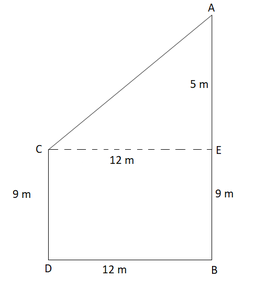We have,

AC = 14 m. DC = 12m and ED = BC = 9 m

Construction: Draw EB ⊥ AC

AB = AC –  BC = 14 — 9 = 5 m

And, EB = DC = 12m

In ∆ABE,

By using Pythagoras theorem

AE2 = AB2 + BE2

⟹ AE2 = 52 + 122

⟹ AE2 = 25 + 144 = 169

⟹ AE= √169 = 13 m

Hence, the distance between their tops = 13 m

### Question 9. Using Pythagoras theorem determine the length of AD in terms of b and c shown in the below fig.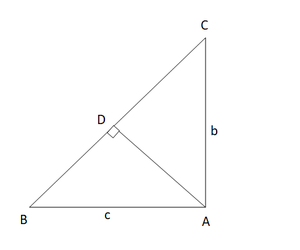Solution:

We have,

In ∆BAC,

By using Pythagoras theorem

BC2 = AB2 + AC2

⟹ BC2 = c2 + b2….(1)

In ∆ABD and ∆CBA

∠B = ∠B                                           [Common]

So, by AA both the triangles are similar

∆ABD ͏~ ∆CBA

Hence, by corresponding parts of similar ∆ are proportionalHence, the length of AD in terms of b and c is### Question 10. A triangle has sides 5 cm, 12 cm, and 13 cm. Find the length to one decimal place, of the perpendicular from the opposite vertex to the side whose length is 13 cm.

Solution: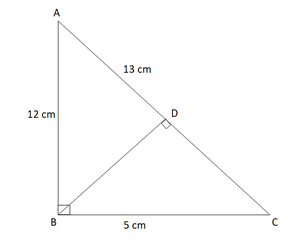Let, AB = 5cm, BC = 12 cm and AC = 13 cm. Then, AC2 = AB2 + BC2

This proves that ∆ABC is a fight triangle. right angles at B.

Let BD be the length of perpendicular from B on AC.

Now, Area of ∆ABC = 1/2(BC x BA)

= 1/2(12 x 5)

= 30 cm

Also, Area of ∆ABC = 1/2(AC x BD) = 1/2(13 x BD) = 1/2(13 x 2)

BD = 60/13 cm

### Question 11. ABCD is a square, F is the mid-point of AB. BE is one third of BC. If the area of ∆FBE = 108 cm2 find the length of AC.

Solution: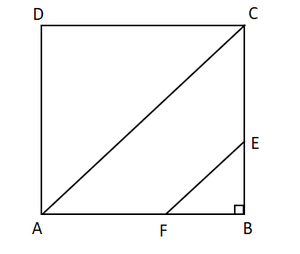Given,

ABCD is a square.

F is the mid-point of AB.

BE is one third of BC.

Area of ∆ FBE = 108cm2

To find: length of AC

Let’s the sides of the square to be y.

AB = BC = CD = DA = y cm

So, AF = FB = x/2 cm

and, BE = x/3 cm

Now,

Area of ∆ FBE = 1/2 x BE x FB

⇒ 108 = (1/2) x (x/3) x (x/2)

⇒ x2 = 108 x 2 x 3 x 2 = 1296

⇒ x = √(1296)

x = 36cm

In ∆ ABC,

By using Pythagoras theorem

AC2 = AB2 + BC

⇒ AC2 = x2 + x2 = 2x2

⇒ AC2 = 2 x (36)2

⇒ AC = 36√2 = 36 x 1.414 = 50.904 cm

Hence, the length of AC is 50.904 cm.

### Question 12. In an isosceles triangle ABC, if AB = AC = 13 cm and the altitude from A on BC is 5 cm, find BC.

Solution: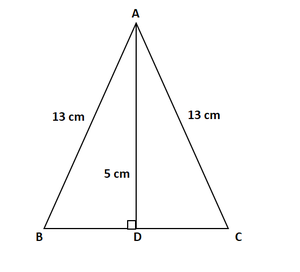Given that,

An isosceles triangle ABC, AB = AC = 13cm, AD = 5cm

To find: BC

By using Pythagoras theorem

52 + BD2 = 169

BD2 = 169 – 25 = 144

BD = √144 = 12 cm

By using Pythagoras theorem

132 = 52 + DC2

169 – 25 = DC2

DC = √144 = 12 cm

So, BC = BD + DC = 12 + 12 = 24 cm

Hence, in isosceles triangle ABC side BC is 24 cm

### Question 13. In a ∆ABC, AB = BC = CA = 2a and AD ⊥ BC. Prove that

(ii) area (∆ABC) = √3 a2

Solution: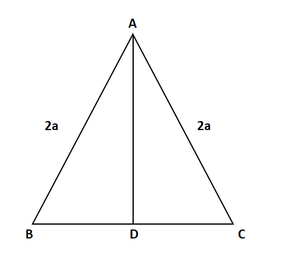(i) In ∆ABD and ∆ACD,

AB = AC                                [Given]

So, By RHS condition

∆ABD ≅ ∆ACD

Hence, by corresponding parts of congruent triangles

∴ BD = CD = a

Now In ∆ABD,

By using Pythagoras theorem

AD2 = 4a2 – a2 = 3a2

(ii) Area (∆ABC) = 1/2 x BC x AD

= 1/2 x (2a) x (a√3)

= √3 a2

Hence Proved

### Question 14. The lengths of the diagonals of a rhombus are 24 cm and 10 cm. Find each side of the rhombus.

Solution: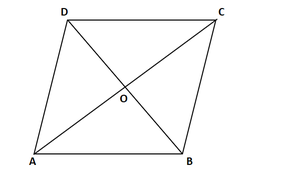To find: Each side of the rhombus.

Let ABCD be a rhombus with diagonals AC and BD.

∴ AC = 24cm and BD = 10cm

We know that,

AO = OC = 12cm and BO = OD = 3cm   [Diagonals of a rhombus bisect each other at right angle]

Now In ∆AOB,

By using Pythagoras theorem

AB2 = AO2 + BO2

= 122 + 52

= 144 + 25

= 169

∴ AB = √(169) = 13cm

∴ AB = BC = CD = AD = 13cm.    [The sides of rhombus are all equal.]

Hence, the sides of the rhombus are as follows AB = BC = CD = AD = 13cm.

Whether you're preparing for your first job interview or aiming to upskill in this ever-evolving tech landscape, GeeksforGeeks Courses are your key to success. We provide top-quality content at affordable prices, all geared towards accelerating your growth in a time-bound manner. Join the millions we've already empowered, and we're here to do the same for you. Don't miss out - check it out now!

Related Tutorials